Profilers > Simulator > Statistical Details for the Simulator
Publication date: 11/29/2021

# Statistical Details for the Simulator

## Normal Weighted Distribution

JMP uses the multivariate radial strata method for each factor that uses the Normal Weighted distribution. This seems to work better than a number of importance sampling methods and is accurate at estimating in the extreme tails.

First, define the number of strata. The strata are a net of hyperspheres that are centered around 0. For d random factors, the strata are defined by their radial intervals. See Table 8.1.

Table 8.1 Strata Intervals

Strata Number

Inside Distance

Outside Distance

0

0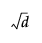1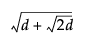2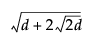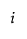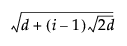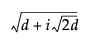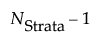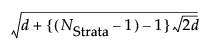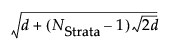The default number of strata is 16. To change the number of strata, a hidden command N Strata is available if you hold the Shift key down while clicking on the red triangle next to Simulator. Increase the sample size as needed to maintain an even number of strata.

For each simulation run, the following is done:

1. Select a strata as mod(i – 1, NStrata) for run i.

2. Determine a random n-dimensional direction by scaling multivariate Normal (0,1) deviates to unit norm.

3. Determine a random distance using a chi-square quantile appropriate for the strata of a random uniform argument.

4. Scale the variates so that the norm is the random distance.

5. Scale and re-center the variates individually to be as specified for each factor.

The resulting factor distributions are multivariate normal with the appropriate means and standard deviations when estimated with the right weights. Note that you cannot use the Distribution standard deviation with weights, because it does not estimate the desired value. However, multiplying the weight by a large value, like 1012, and using that as a Freq value results in the correct standard deviation.